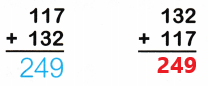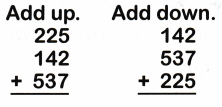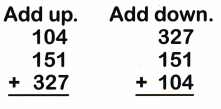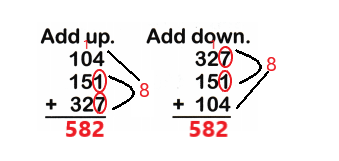# McGraw Hill Math Grade 2 Chapter 5 Lesson 6 Answer Key Adding Three-Digit Numbers in Any Order or Group

Practice questions available in McGraw Hill Math Grade 2 Answer Key PDF Chapter 5 Lesson 6 Adding Three-Digit Numbers in Any Order or Group will engage students and is a great way of informal assessment.

## McGraw-Hill Math Grade 2 Answer Key Chapter 5 Lesson 6 Adding Three-Digit Numbers in Any Order or Group

write the sum.

Question 1.Explanation:
The order of the addends may differ but the sum will be same
So, The sum of 117 and 132 is 249.

Question 2.Explanation:
The order of the addends may differ but the sum will be same
So, The sum of 289 and 537 is 826.

Question 3.Explanation:
The order of the addends may differ but the sum will be same
We can add going up or going down
We should group the numbers we add
So, the sum of 225, 142 and 537 is 904.

Question 4.